# Resources tagged with: Maths Supporting SET

Filter by: Content type:
Age range:
Challenge level:

### There are 90 results

Broad Topics > Cross-curricular Contexts > Maths Supporting SET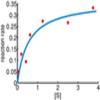### Curve Fitter 2

##### Age 16 to 18 Challenge Level:

Can you construct a cubic equation with a certain distance between its turning points?### Integration Matcher

##### Age 16 to 18 Challenge Level:

Can you match the charts of these functions to the charts of their integrals?### Scientific Curves

##### Age 16 to 18 Challenge Level:

Can you sketch these difficult curves, which have uses in mathematical modelling?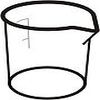### Brimful

##### Age 16 to 18 Challenge Level:

Can you find the volumes of the mathematical vessels?### Equation Matcher

##### Age 16 to 18 Challenge Level:

Can you match these equations to these graphs?### Population Dynamics Collection

##### Age 16 to 18 Challenge Level:

This is our collection of tasks on the mathematical theme of 'Population Dynamics' for advanced students and those interested in mathematical modelling.### Maths Filler 2

##### Age 14 to 16 Challenge Level:

Can you draw the height-time chart as this complicated vessel fills with water?### Real-life Equations

##### Age 16 to 18 Challenge Level:

Here are several equations from real life. Can you work out which measurements are possible from each equation?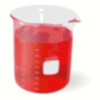### Brimful 2

##### Age 16 to 18 Challenge Level:

Which of these infinitely deep vessels will eventually full up?### What's That Graph?

##### Age 14 to 16 Challenge Level:

Can you work out which processes are represented by the graphs?### Immersion

##### Age 14 to 16 Challenge Level:

Various solids are lowered into a beaker of water. How does the water level rise in each case?### Guessing the Graph

##### Age 14 to 16 Challenge Level:

Can you suggest a curve to fit some experimental data? Can you work out where the data might have come from?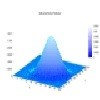### Pdf Matcher

##### Age 16 to 18 Challenge Level:

Which pdfs match the curves?### Debt Race

##### Age 16 to 18 Challenge Level:

Who will be the first investor to pay off their debt?### Reaction Rates

##### Age 16 to 18 Challenge Level:

Explore the possibilities for reaction rates versus concentrations with this non-linear differential equation### Square Pair

##### Age 16 to 18 Challenge Level:

Explore the shape of a square after it is transformed by the action of a matrix.### Whose Line Graph Is it Anyway?

##### Age 16 to 18 Challenge Level:

Which line graph, equations and physical processes go together?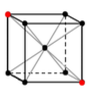### Crystal Symmetry

##### Age 16 to 18 Challenge Level:

Use vectors and matrices to explore the symmetries of crystals.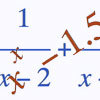### Root Hunter

##### Age 16 to 18 Challenge Level:

In this short problem, try to find the location of the roots of some unusual functions by finding where they change sign.### Differential Equation Matcher

##### Age 16 to 18 Challenge Level:

Match the descriptions of physical processes to these differential equations.### Operating Machines

##### Age 16 to 18 Challenge Level:

What functions can you make using the function machines RECIPROCAL and PRODUCT and the operator machines DIFF and INT?### Spherical Triangles on Very Big Spheres

##### Age 16 to 18 Challenge Level:

Shows that Pythagoras for Spherical Triangles reduces to Pythagoras's Theorem in the plane when the triangles are small relative to the radius of the sphere.### Transformations for 10

##### Age 16 to 18 Challenge Level:

Explore the properties of matrix transformations with these 10 stimulating questions.### Flight Path

##### Age 16 to 18 Challenge Level:

Use simple trigonometry to calculate the distance along the flight path from London to Sydney.##### Age 14 to 18 Challenge Level:

How would you design the tiering of seats in a stadium so that all spectators have a good view?### Back Fitter

##### Age 14 to 16 Challenge Level:

10 graphs of experimental data are given. Can you use a spreadsheet to find algebraic graphs which match them closely, and thus discover the formulae most likely to govern the underlying processes?### Far Horizon

##### Age 14 to 16 Challenge Level:

An observer is on top of a lighthouse. How far from the foot of the lighthouse is the horizon that the observer can see?### Ball Bearings

##### Age 16 to 18 Challenge Level:

If a is the radius of the axle, b the radius of each ball-bearing, and c the radius of the hub, why does the number of ball bearings n determine the ratio c/a? Find a formula for c/a in terms of n.### Robot Camera

##### Age 14 to 16 Challenge Level:

Could nanotechnology be used to see if an artery is blocked? Or is this just science fiction?### Polygon Walk

##### Age 16 to 18 Challenge Level:

Go on a vector walk and determine which points on the walk are closest to the origin.### Nine Eigen

##### Age 16 to 18 Challenge Level:

Explore how matrices can fix vectors and vector directions.### Perfect Eclipse

##### Age 14 to 16 Challenge Level:

Use trigonometry to determine whether solar eclipses on earth can be perfect.### Matrix Meaning

##### Age 16 to 18 Challenge Level:

Explore the meaning behind the algebra and geometry of matrices with these 10 individual problems.### Speed-time Problems at the Olympics

##### Age 14 to 16 Challenge Level:

Have you ever wondered what it would be like to race against Usain Bolt?### Big and Small Numbers in Chemistry

##### Age 14 to 16 Challenge Level:

Get some practice using big and small numbers in chemistry.### Pdf Stories

##### Age 16 to 18 Challenge Level:

Invent scenarios which would give rise to these probability density functions.### 3D Drawing

##### Age 11 to 16

The design technology curriculum requires students to be able to represent 3-dimensional objects on paper. This article introduces some of the mathematical ideas which underlie such methods.### Air Nets

##### Age 7 to 18 Challenge Level:

Can you visualise whether these nets fold up into 3D shapes? Watch the videos each time to see if you were correct.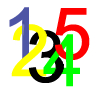### Track Design

##### Age 14 to 16 Challenge Level:

Where should runners start the 200m race so that they have all run the same distance by the finish?### Perspective Drawing

##### Age 11 to 16 Challenge Level:

Explore the properties of perspective drawing.### Investigating the Dilution Series

##### Age 14 to 16 Challenge Level:

Which dilutions can you make using only 10ml pipettes?### Truth Tables and Electronic Circuits

##### Age 11 to 18

Investigate circuits and record your findings in this simple introduction to truth tables and logic.### What Do Functions Do for Tiny X?

##### Age 16 to 18 Challenge Level:

Looking at small values of functions. Motivating the existence of the Taylor expansion.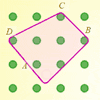### Elastic Maths

##### Age 14 to 18

How do you write a computer program that creates the illusion of stretching elastic bands between pegs of a Geoboard? The answer contains some surprising mathematics.### Witch's Hat

##### Age 11 to 16 Challenge Level:

What shapes should Elly cut out to make a witch's hat? How can she make a taller hat?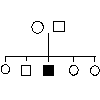### Genetics

##### Age 14 to 16 Challenge Level:

A problem about genetics and the transmission of disease.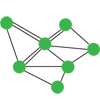### Investigating Epidemics

##### Age 11 to 16 Challenge Level:

Simple models which help us to investigate how epidemics grow and die out.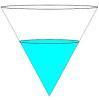### Fill Me up Too

##### Age 14 to 16 Challenge Level:

In Fill Me Up we invited you to sketch graphs as vessels are filled with water. Can you work out the equations of the graphs?### Designing Table Mats

##### Age 11 to 16 Challenge Level:

Formulate and investigate a simple mathematical model for the design of a table mat.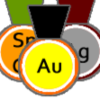### Alternative Record Book

##### Age 14 to 18 Challenge Level:

In which Olympic event does a human travel fastest? Decide which events to include in your Alternative Record Book.